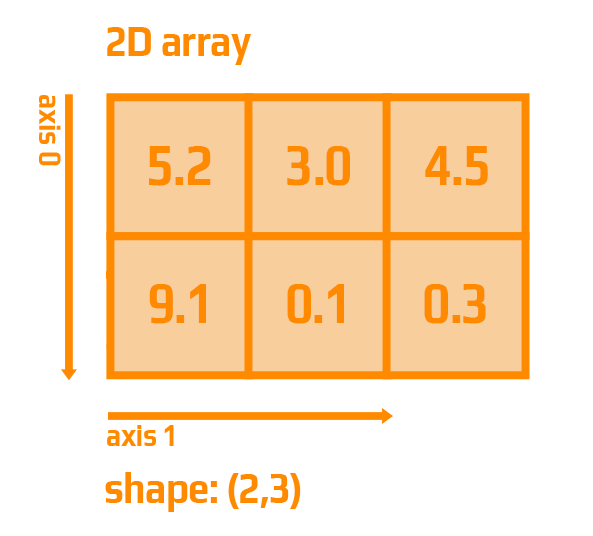Course Content

# NumPy in a Nutshell

NumPy in a Nutshell

##2-D Arrays

Now we have moved on to the most interesting thing! What is a two-dimensional array?

In the general case, two-dimensional arrays are called matrices: such mathematical structures that consist of rows and columns. If it will be easier for you, then you can represent the matrix as a table.

Now is the time to practice with 2D arrays. Let's take a look how to create a 2-D array which contain such two arrays as `5.2, 3.0, 4.5`, and `9.1, 0.1, 0.3`:#Select all options with the INCORRECT code of creating 2-D arrays.

## Select a few correct answers

Everything was clear?

Section 2. Chapter 6